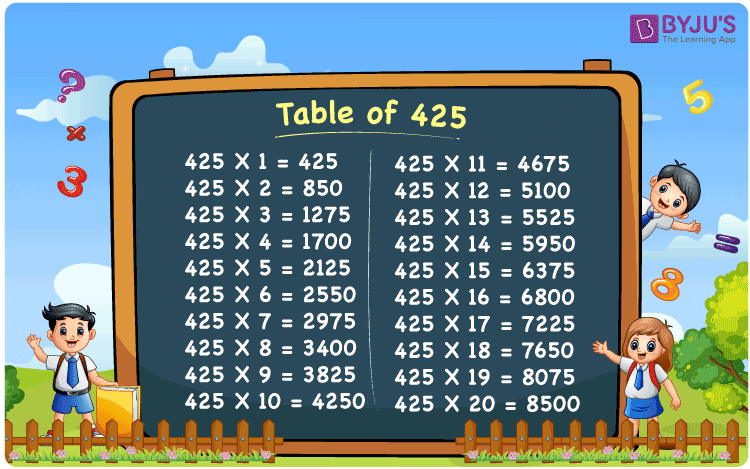Checkout JEE MAINS 2022 Question Paper Analysis : Checkout JEE MAINS 2022 Question Paper Analysis :

# Table of 425

In mathematics, the table of 425 is the multiplication table that shows the product of 425 with consecutive natural numbers. 425 times table can be produced by repeated addition of the number to itself. Table of 425 up to 20 times is available here to help students solve arithmetic problems. Learn more about Multiplication tables at BYJU’S and download the PDF of table 425 here for free.

## Table of 425 Chart## What is 425 Times Table?

The table below shows the repeated addition of the number 425 to get the required multiple.

 425×1 = 425 425 425×2 = 850 425 + 425 = 850 425×3 = 1275 425 + 425 + 425 = 1275 425×4 = 1700 425 + 425 + 425 + 425 = 1700 425×5 = 2125 425 + 425 + 425 + 425 + 425 = 2125 425×6 = 2550 425 + 425 + 425 + 425 + 425 + 425 = 2550 425×7 = 2975 425 + 425 + 425 + 425 + 425 + 425 + 425 = 2975 425×8 = 3400 425 + 425 + 425 + 425 + 425 + 425 + 425 + 425 = 3400 425×9 = 3825 425 + 425 + 425 + 425 + 425 + 425 + 425 + 425 + 425 = 3825 425×10 = 4250 425 + 425 + 425 + 425 + 425 + 425 + 425 + 425 + 425 + 425 = 4250

## Multiplication Table of 425

Here is the table of 425 up to 20 times below.

 425 × 1 = 425 425 × 2 = 850 425 × 3 = 1275 425 × 4 = 1700 425 × 5 = 2125 425 × 6 = 2550 425 × 7 = 2975 425 × 8 = 3400 425 × 9 = 3825 425 × 10 = 4250 425 × 11 = 4675 425 × 12 = 5100 425 × 13 = 5525 425 × 14 = 5950 425 × 15 = 6375 425 × 16 = 6800 425 × 17 = 7225 425 × 18 = 7650 425 × 19 = 8075 425 × 20 = 8500

## Solved Example on Table of 425

Evaluate: 425 x 3 – 500

Solution: Given,

425 x 3 – 500

From the table of 425, we have;

425 x 3 = 1275

Therefore,

1275 – 500 = 775

## Frequently Asked Questions on Table of 425

### What is table of 425 in Maths?

Table of 425 represents the multiples of the original number when 425 is multiplied by a set of positive integers.

### What is the value of 425 multiplied by 4?

The value of 425 multiplied by 4 is equal to 1700.

### Does 3400 come in table of 425?

Yes , 3400 comes in table of 425 since 425 x 8 = 3400.